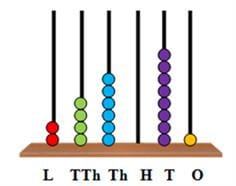Test: Introduction to Numbers- 1

# Test: Introduction to Numbers- 1

Test Description

## 10 Questions MCQ Test Mathematics for Class 4 | Test: Introduction to Numbers- 1

Test: Introduction to Numbers- 1 for Class 4 2023 is part of Mathematics for Class 4 preparation. The Test: Introduction to Numbers- 1 questions and answers have been prepared according to the Class 4 exam syllabus.The Test: Introduction to Numbers- 1 MCQs are made for Class 4 2023 Exam. Find important definitions, questions, notes, meanings, examples, exercises, MCQs and online tests for Test: Introduction to Numbers- 1 below.
Solutions of Test: Introduction to Numbers- 1 questions in English are available as part of our Mathematics for Class 4 for Class 4 & Test: Introduction to Numbers- 1 solutions in Hindi for Mathematics for Class 4 course. Download more important topics, notes, lectures and mock test series for Class 4 Exam by signing up for free. Attempt Test: Introduction to Numbers- 1 | 10 questions in 15 minutes | Mock test for Class 4 preparation | Free important questions MCQ to study Mathematics for Class 4 for Class 4 Exam | Download free PDF with solutions
 1 Crore+ students have signed up on EduRev. Have you?
Test: Introduction to Numbers- 1 - Question 1

### Which of the following is true for the number 82,951?

Detailed Solution for Test: Introduction to Numbers- 1 - Question 1

The statement ‘2 is the face value of 2 in 82,951’ is true.
The false statements can be corrected as 8 is the face value of 8 in 82,951.
900 is the place value of 9 in 82,951.
50 is the place value of 5 in 82,951.

Test: Introduction to Numbers- 1 - Question 2

### Which of the following is the smallest 5-digit number?

Detailed Solution for Test: Introduction to Numbers- 1 - Question 2

The number 10,000 is the smallest 5-digit number.
It comes just after the greatest 4-digit number 9999.

Test: Introduction to Numbers- 1 - Question 3

### What is the place value of the underlined digit in the number 7,65,730?

Detailed Solution for Test: Introduction to Numbers- 1 - Question 3

The place value of a number is the value of the digit in the given number.
The place value of the underlined digit in the number 7,65,730 is 7,00,000 as it is in the lakhs place.

Test: Introduction to Numbers- 1 - Question 4

Which of the following shows the first three places from the right in a 5-digit number?

Detailed Solution for Test: Introduction to Numbers- 1 - Question 4

In a 5-digit number, the first three places from the right are the ones, tens, and hundreds places. These make up the ones period. The thousands period consists of the thousands and ten thousands places, which are the fourth and fifth places from the right

Test: Introduction to Numbers- 1 - Question 5

Which of the following can also be written as 4,50,000?

Detailed Solution for Test: Introduction to Numbers- 1 - Question 5

There are 45 ten thousands in 4,50,000.
Hence, it can also be written as forty-five ten thousands.

Test: Introduction to Numbers- 1 - Question 6

One lakh can be expressed as ____________.

Detailed Solution for Test: Introduction to Numbers- 1 - Question 6

1,00,000 is equal to 100 thousands.
1,00,000 is read as one lakh.
Hence, 1,00,000 can be expressed as 100 thousands.

Test: Introduction to Numbers- 1 - Question 7

Which of the following options is read as thirty-five thousand six hundred and thirty-five?

Detailed Solution for Test: Introduction to Numbers- 1 - Question 7

There are three ten thousands, five thousands, six hundreds, three tens and five ones in the number 35,635. Hence, we read 35,635 as thirty-five thousand six hundred and thirty-five.

Test: Introduction to Numbers- 1 - Question 8

Which of the following is the standard form for the given expanded form? 40,000 + 6,000 + 700 + 80 + 1

Detailed Solution for Test: Introduction to Numbers- 1 - Question 8

In the standard form, we write 40,000 + 6,000 + 700 + 80 + 1 as 46,781.

Test: Introduction to Numbers- 1 - Question 9

Which of the following is one more than 99,999?

Detailed Solution for Test: Introduction to Numbers- 1 - Question 9

99,999 is the greatest 5-digit number.
When 1 is added to 99,999, we get the 6-digit number 1,00,000.

Test: Introduction to Numbers- 1 - Question 10

Which of the following is the number which can be represented using the given abacus?Detailed Solution for Test: Introduction to Numbers- 1 - Question 10

There are 2 beads in the lakhs place, 4 beads in the ten thousands place, 6 beads in the thousands place, 0 beads in the hundreds place, eight beads in the tens place and one bead in the ones place.
Hence, the number on the abacus is 2,46,081.

## Mathematics for Class 4

14 videos|68 docs|32 tests
Information about Test: Introduction to Numbers- 1 Page
In this test you can find the Exam questions for Test: Introduction to Numbers- 1 solved & explained in the simplest way possible. Besides giving Questions and answers for Test: Introduction to Numbers- 1, EduRev gives you an ample number of Online tests for practice

## Mathematics for Class 4

14 videos|68 docs|32 tests(Scan QR code)